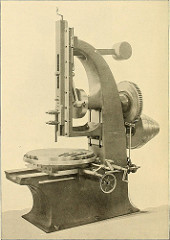#Math, Statistics, and Engineering

In college, Mechanics was a required class from the civil engineering department. This included differential equation.

Luckily for me, I also enjoyed a required course called analytical mechanics for my physics degree. This included using Lagrange and Hamiltonian equations to derived a wide range of formulas to solve mechanisms problems.

In the civil engineering course, the professor did the derivation as the course lectures, then expected us to use the right formula to solve a problem. He even gave us a ‘cheat sheet’ with an assortment of derived equations. We just had to identify which equation to use for a particular problem and ‘plug-and-chug’ or just work out the math. It was boring. Continue reading Math, Statistics, and Engineering